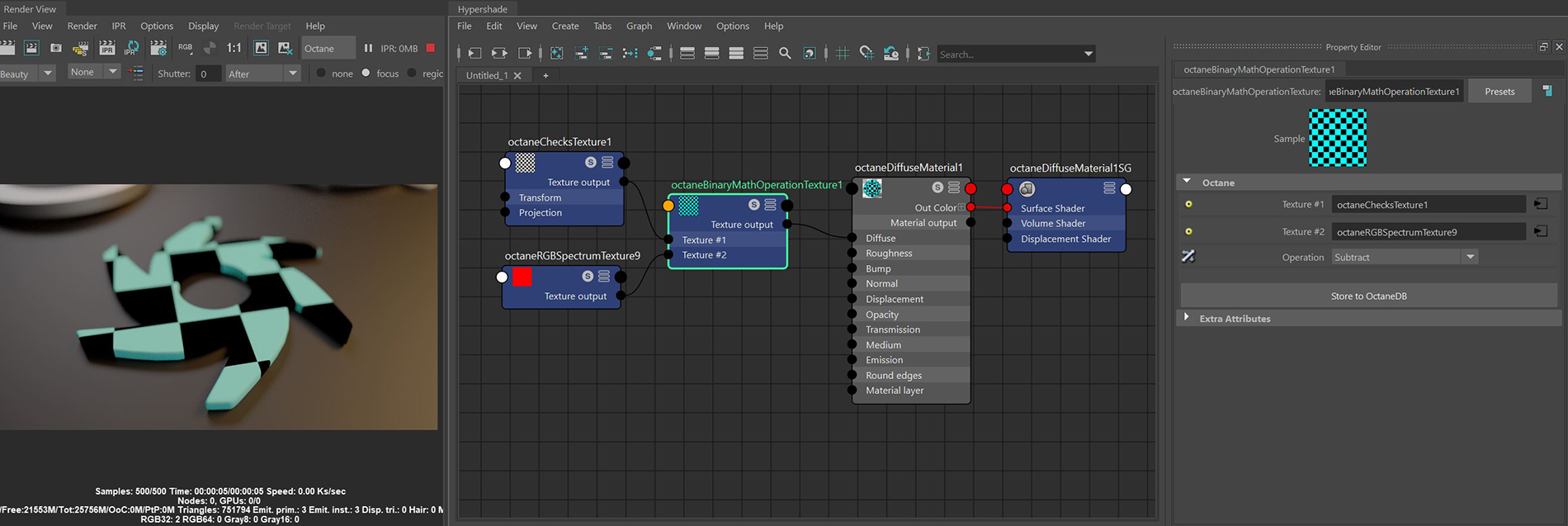# Binary Math Operation Texture

The Binary Math node takes two inputs as operands for various mathematical calculations determined in the Operation parameter (figure 1).Figure 1: A Checks and a RGB Spectrum node are blended together using the Subtract Operation

### Binary Math Operation Texture Attributes

Argument A - The first argument for the operation.

Argument B - The second argument for the operation.

Operation - The calculation to be performed on the arguments.